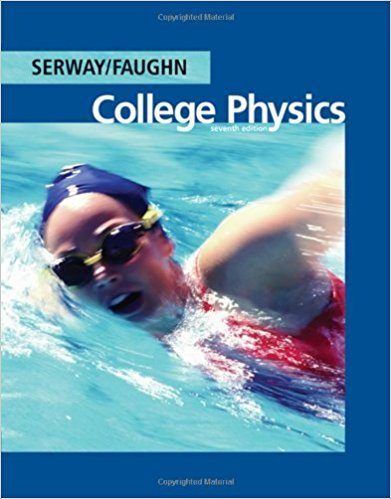×
Get Full Access to College Physics - 7 Edition - Chapter 13 - Problem 13.6
Get Full Access to College Physics - 7 Edition - Chapter 13 - Problem 13.6

×

# An archer must exert a force of 375 N on the bowstringISBN: 9780495113690 154

## Solution for problem 13.6 Chapter 13

College Physics | 7th Edition

• Textbook Solutions
• 2901 Step-by-step solutions solved by professors and subject experts
• Get 24/7 help from StudySoup virtual teaching assistantsCollege Physics | 7th Edition

4 5 1 245 Reviews
21
4
Problem 13.6

An archer must exert a force of 375 N on the bowstring shown in Figure P13.6a (page 452) such that the string makes an angle of 35.0 with the vertical. (a) Determine the tension in the bowstring. (b) If the applied force is replaced by a stretched spring as in Figure P13.6b, and the spring is stretched 30.0 cm from its unstretched length, what is the spring constant?

Step-by-Step Solution:
Step 1 of 3

Modern Physics Lecture 1: Foundations and Special Relativity Position, Time, and Rotation are all continuous symmetries (discrete symmetries such as reflection do not produce the same results as below):  Invariance in position (changing where in space you perform your experiment) proves momentum conservation  Invariance in rotation (eg. rotating your entire experiment by 90 degrees) proves conservation of angular momentum  Invariance in time (changing when in time you perform your experiment, eg. today vs. tomorrow) proves energy conservation Newtonian Mechanics:  Inertia  F=ma  The force of object 1 on object 2 is equal in magnitude to the force of object 2 on object 1  True in an inertial reference frame

Step 2 of 3

Step 3 of 3

##### ISBN: 9780495113690

Unlock Textbook Solution